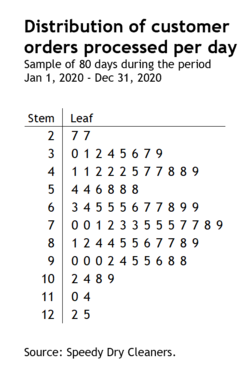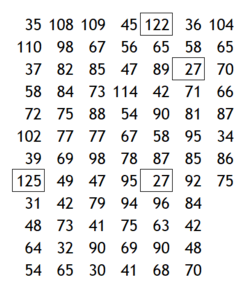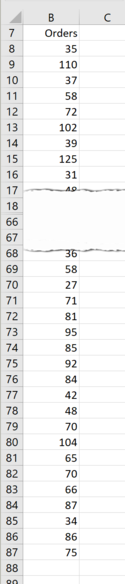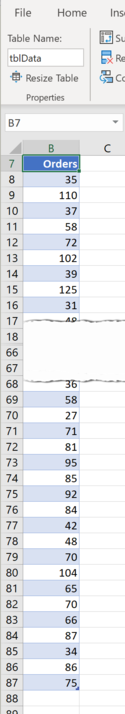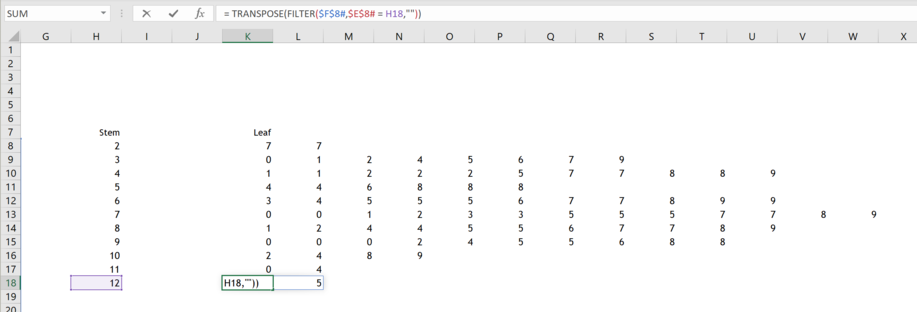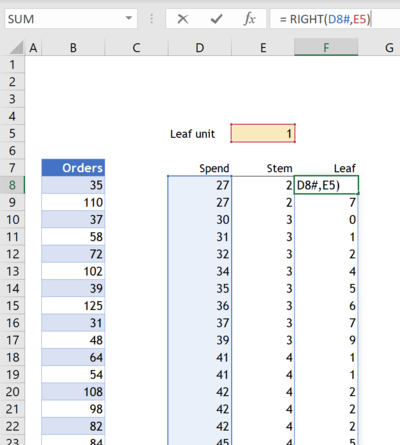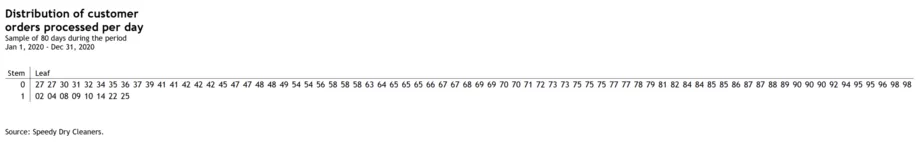• WORK
• TOOLS
• TRAINING
• VIEWS
• CONTACT

A Stem-and-Leaf plot is an alternative way to show data distributions. This approach is similar to the histogram except that the actual data are displayed instead of the bars.

The chart below illustrates the basic idea of a Stem-and-Leaf plot. It shows the number of customer orders processed per day for Speedy Dry Cleaners—for a sample of 80 randomly selected days.

# HOW TO CREATE A STEM-AND-LEAF PLOT IN EXCEL

Lets turn the data source into an Excel Table.

1. Select a cell within the data.
2. Press Ctrl + T to open the Create Table dialog box. Press the Ok button to close the Create Table dialog box.
3. In the Properties group, under the Table Design tab, type a new table name (i.e. tblData) in the Table Name box and press Enter.
4. Next, uncheck the Filter Button in the Table Style Options group.
The data should look like the snapshot below

The first step in the creation of the Stem-and-Leaf plot is to sort the data from smallest to largest. To do that I'm going to use the new SORT function—part of Excel's new Dynamic Arrays functionality.

5. Type the following formula in cell D8:
= SORT(tblData[Orders])

Next, I need to identify the leaf and stem for each entry in the table, and that depends on the leaf unit used.

6. Enter the label "Leaf unit" in cell D5 and the value 1 in cell E5.
7. Type the following formula in cell E8:
= IFERROR(VALUE(LEFT(D8#,LEN(D8#) - E5)),0)
8. Type the following formula in cell F8:
= RIGHT(D8#,E5)

The worksheet should look like this:

9. Type the following formula in cell H8:
= SEQUENCE(MAX(E8#) - MIN(E8#) + 1,1,MIN(E8#),1)
10. Type the following formula in cell K8:
= TRANSPOSE(FILTER(\$F\$8#,\$E\$8# = H8,""))
11. Select cell K8 and drag the handle of the cell to copy the formula into the range K9:K18.
The worksheet should look like this:

12. Change the width of columns H-X, add the vertical and horizontal lines to separate the stem from the leaf by formatting the cell borders, add the chart title and source. The chart should look like this:

13. Finally, you'll notice that the chart is dynamic. Change the leaf unit to 2 and the chart should look like this:

Each individual value from the sample of 80 entries (top right) is recorded in the Stem-and-Leaf plot as a combination of two parts—The stem and the leaf. Depending on the choice of the leaf unit—in our case 1—we treat the first digit (the 10s place) as the stem and the second digit (the 1s place) as the leaf. A leaf unit of 1 implies a binning on the stem in 10s, that is, 20, 30, 40, all the way to 110 and 120.

For example the smallest value in the sample is 27. This is read in the Stem-and-Leaf plot on the first row by combining the stem digit "2" and the leaf digit "7". Because we have two "27" entries in the sample in the range 20-30-, we read two "7" in the leaf.

Similarly, the largest number in the sample is 125. This is read on the last row of the chart by combining the stem digit "12" and the leaf digit "5". The second largest number is "122", hence the leaf digit is "2".

Lets build the chart in Excel. The data is in the range B7:B87.

Views > Excel charts > How to create a stem-and-leaf plot in excel

HOW TO CREATE A STEM-AND-LEAF PLOT IN EXCEL

Thank you for reading, and I hope you found this useful. If you have any questions, find me on Twitter and ask me anything.